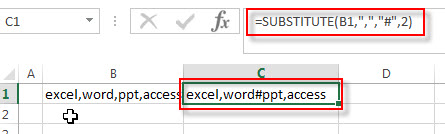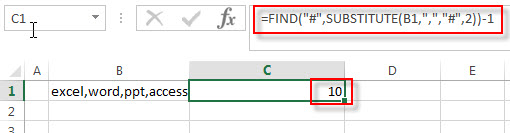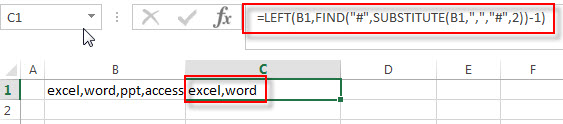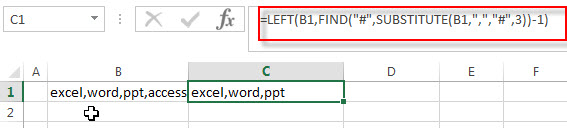# How to extract text before the second or nth specific character (space or comma)

Before we talked that how to extract text before the first space or comma character in excel. And this post will guide you how to extract a substring before the second or nth specific character, such as: space or comma character in excel.

## Extract text before the second or nth specific character

If you want to extract a substring before the second or nth match of the comma character from a text string in Cell b1, and you need to know the position of the second occurrence of the comma character in text firstly, so you can use the SUBSTITUTE function to lookup the second comma and replace it with hash character, then use FIND function or SEARCH function to search the hash character in text that returned by the SUBSTITUTE function, and the returned value of the FIND function is the position of the second commas in text.Last, you can use the LEFT function to extract the leftmost characters before the position of the second comma.

So you can create a formula based on the LEFT function, the FIND function and the SUBSTITUTE function as follows:

=LEFT(B1,FIND(“#”,SUBSTITUTE(B1,”,”,”#”,2))-1)

Where the 2 number represents the comma instance number, and if you want to extract the nth match of the comma character, you need to replace number 2 to another number that you need.

Let’s see how this formula works:

=SUBSTITUTE(B1,”,”,”#”,2)This function replaces the second comma character with hash character in cell B1, and the returned result goes into the FIND function as its within_text argument.

=FIND(“#”,SUBSTITUTE(B1,”,”,”#”,2))-1You have got the within_text from the above SUBSTITUTE function and the FIND function will return the position of the first occurrence of the hash character in within_text string. Then subtract 1 to get the position before the second occurrence of the comma character in text. And it returns 10. It is fed into the LEFT function as its num_chars argument.

=LEFT(B1,FIND(“#”,SUBSTITUTE(B1,”,”,”#”,2))-1)This formula extract the leftmost 10 characters from a text string in Cell B1, and the returned string is what you want to extract, like this:

`excel,word`

If you want to extract a substring before the third occurrence of the comma character, you can use the following formula:

=LEFT(B1,FIND(“#”,SUBSTITUTE(B1,”,”,”#”,3))-1)If you want to extract a substring before the nth occurrence of the space character or others specific characters, you just need to replace comma symbol to space or other characters that you need.

=LEFT(B1,FIND(“#”,SUBSTITUTE(B1,” “,”#”,Nth))-1)

### Related Formulas

• Extract Text between Parentheses
If you want to extract text between parentheses in a cell, then you can use the search function within the MID function to create a new excel formula…
• Extract Text between Brackets
If you want to extract text between brackets in a cell, you need to create a formula based on the SEARCH function and the MID function….
• Extract Text between Commas
To extract text between commas in Cell B1, you can use the following formula based on the SUBSTITUTE function, the MID function and the REPT function…..
• Extract word that starting with a specific character
Assuming that you have a text string that contains email address in Cell B1, and if you want to extract word that begins with a specific character “@” sign, you can use a combination with the TRIM function, the LEFT function, the SUBSTITUTE function ….
• Extract text before first comma or space
If you want to extract text before the first comma or space character in cell B1, you can use a combination of the LEFT function and FIND function….
• Extract text after first comma or space
If you want to get substring after the first comma character from a text string in Cell B1, then you can create a formula based on the MID function and FIND function or SEARCH function ….

### Related Functions

• Excel LEFT function
The Excel LEFT function returns a substring (a specified number of the characters) from a text string, starting from the leftmost character.The syntax of the LEFT function is as below:= LEFT(text,[num_chars])….
• Excel FIND function
The Excel FIND function returns the position of the first text string (sub string) within another text string.The syntax of the FIND function is as below:= FIND(find_text, within_text,[start_num])…
• Excel SEARCH function
The Excel SEARCH function returns the number of the starting location of a substring in a text string.The syntax of the SEARCH function is as below:= SEARCH  (find_text, within_text,[start_num])…
• Excel Substitute function
The Excel SUBSTITUTE function replaces a new text string for an old text string in a text string.The syntax of the SUBSTITUTE function is as below:= SUBSTITUTE  (text, old_text, new_text,[instance_num])….